×
Log in to StudySoup
Get Full Access to Probability And Statistical Inference - 9 Edition - Chapter 2.2 - Problem 2.2-1
Join StudySoup for FREE
Get Full Access to Probability And Statistical Inference - 9 Edition - Chapter 2.2 - Problem 2.2-1

Already have an account? Login here
×
Reset your password

# Find E(X) for each of the distributions given in Exercise 2.1-3ISBN: 9780321923271 41

## Solution for problem 2.2-1 Chapter 2.2

Probability and Statistical Inference | 9th Edition

• Textbook Solutions
• 2901 Step-by-step solutions solved by professors and subject experts
• Get 24/7 help from StudySoup virtual teaching assistantsProbability and Statistical Inference | 9th Edition

4 5 1 374 Reviews
16
2
Problem 2.2-1

Find E(X) for each of the distributions given in Exercise 2.1-3.

Step-by-Step Solution:

Problem 2.2-1

Find E(X) for each of the distributions given in Exercise 2.1-3.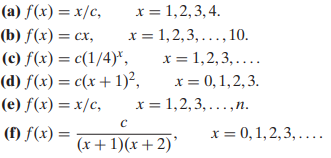Step by Step Solution

Step 1 of 6

(a)

The probability mass function is given as,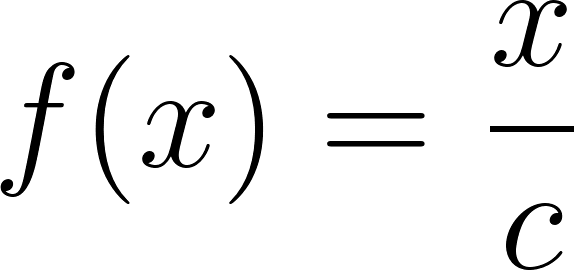where,

x = 1,2,3,4. and  c = 10. (For c value refer solution of Exercise 2.1-3)

For each value of x,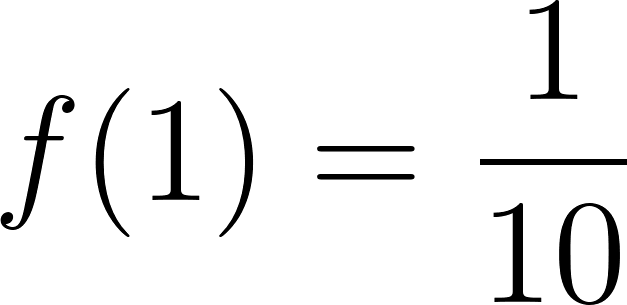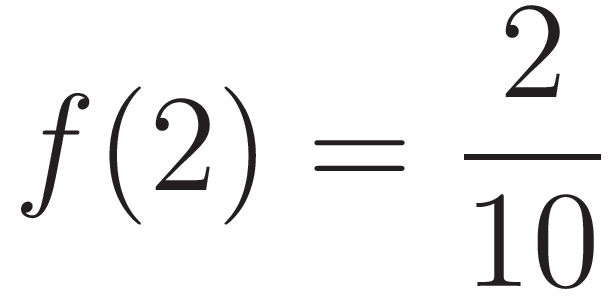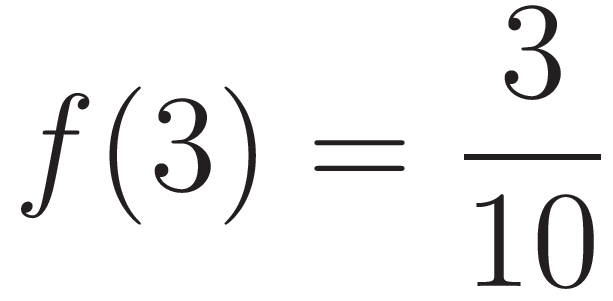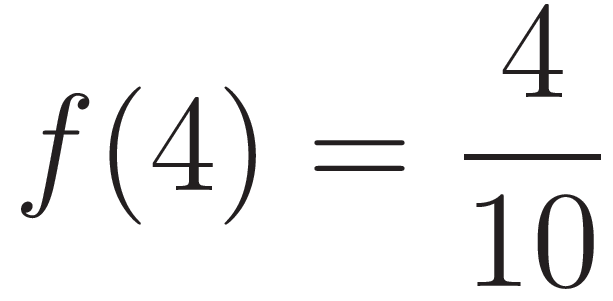The equation for E(X) is give as,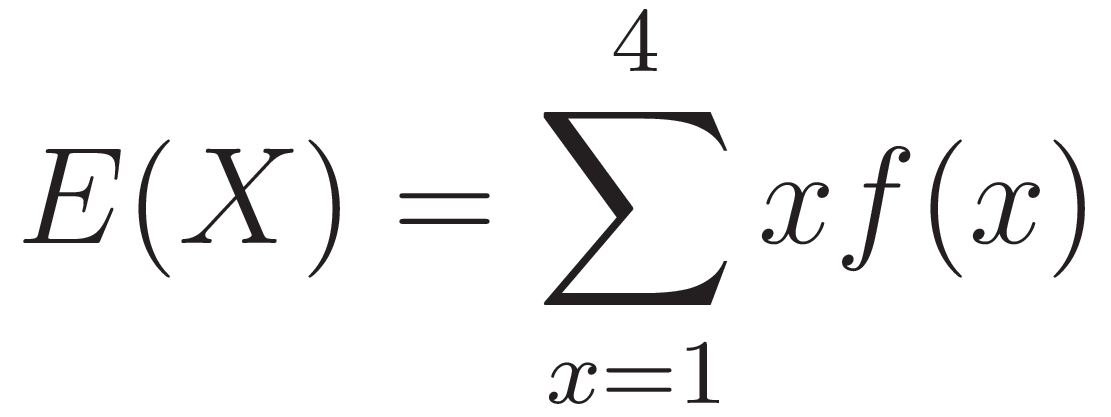The expected value function is the sum of the product of  x value and its pmf value,ie;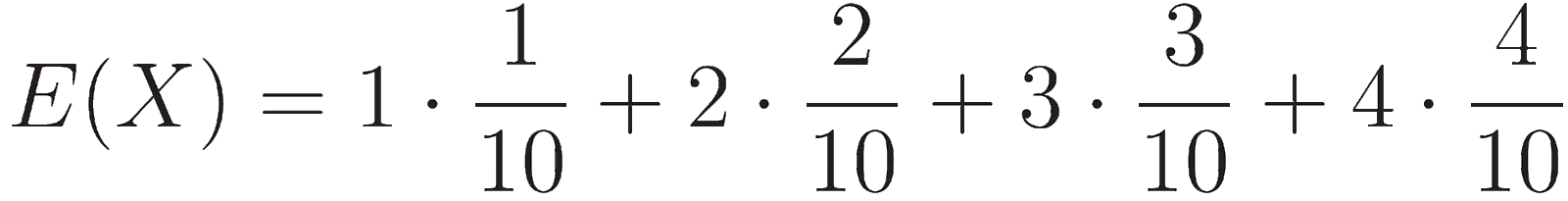Step 2 of 6

Step 3 of 6

##### ISBN: 9780321923271

The full step-by-step solution to problem: 2.2-1 from chapter: 2.2 was answered by , our top Statistics solution expert on 07/05/17, 04:50AM. This full solution covers the following key subjects: . This expansive textbook survival guide covers 59 chapters, and 1476 solutions. This textbook survival guide was created for the textbook: Probability and Statistical Inference , edition: 9. Since the solution to 2.2-1 from 2.2 chapter was answered, more than 557 students have viewed the full step-by-step answer. The answer to “Find E(X) for each of the distributions given in Exercise 2.1-3.” is broken down into a number of easy to follow steps, and 11 words. Probability and Statistical Inference was written by and is associated to the ISBN: 9780321923271.

Unlock Textbook Solution

Enter your email below to unlock your verified solution to:

Find E(X) for each of the distributions given in Exercise 2.1-3